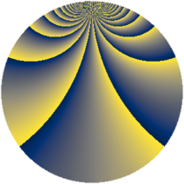# Properties

 Label 805.2.beLevel $805$ Weight $2$ Character orbit 805.be Rep. character $\chi_{805}(29,\cdot)$ Character field $\Q(\zeta_{22})$ Dimension $720$ Newform subspaces $1$ Sturm bound $192$ Trace bound $0$

# Related objects

## Defining parameters

 Level: $$N$$ $$=$$ $$805 = 5 \cdot 7 \cdot 23$$ Weight: $$k$$ $$=$$ $$2$$ Character orbit: $$[\chi]$$ $$=$$ 805.be (of order $$22$$ and degree $$10$$) Character conductor: $$\operatorname{cond}(\chi)$$ $$=$$ $$115$$ Character field: $$\Q(\zeta_{22})$$ Newform subspaces: $$1$$ Sturm bound: $$192$$ Trace bound: $$0$$

## Dimensions

The following table gives the dimensions of various subspaces of $$M_{2}(805, [\chi])$$.

Total New Old
Modular forms 1000 720 280
Cusp forms 920 720 200
Eisenstein series 80 0 80

## Trace form

 $$720q + 80q^{4} + 4q^{5} - 8q^{6} + 64q^{9} + O(q^{10})$$ $$720q + 80q^{4} + 4q^{5} - 8q^{6} + 64q^{9} + 16q^{11} - 8q^{14} - 18q^{15} - 96q^{16} - 36q^{20} + 8q^{21} + 24q^{24} - 30q^{25} + 40q^{26} - 8q^{29} - 44q^{30} - 8q^{31} + 12q^{34} + 4q^{35} - 72q^{36} - 72q^{39} - 20q^{40} - 16q^{41} - 148q^{44} + 32q^{45} - 32q^{46} + 72q^{49} + 32q^{50} - 248q^{54} - 88q^{55} - 72q^{59} - 200q^{60} - 40q^{61} - 20q^{65} + 128q^{66} - 208q^{69} - 8q^{70} + 188q^{71} - 128q^{74} + 132q^{75} - 44q^{76} - 40q^{79} - 180q^{80} - 264q^{81} - 24q^{84} - 4q^{85} - 376q^{86} + 48q^{89} + 394q^{90} - 160q^{91} + 144q^{94} + 64q^{95} - 732q^{96} - 88q^{99} + O(q^{100})$$

## Decomposition of $$S_{2}^{\mathrm{new}}(805, [\chi])$$ into newform subspaces

Label Dim. $$A$$ Field CM Traces $q$-expansion
$$a_2$$ $$a_3$$ $$a_5$$ $$a_7$$
805.2.be.a $$720$$ $$6.428$$ None $$0$$ $$0$$ $$4$$ $$0$$

## Decomposition of $$S_{2}^{\mathrm{old}}(805, [\chi])$$ into lower level spaces

$$S_{2}^{\mathrm{old}}(805, [\chi]) \cong$$ $$S_{2}^{\mathrm{new}}(115, [\chi])$$$$^{\oplus 2}$$